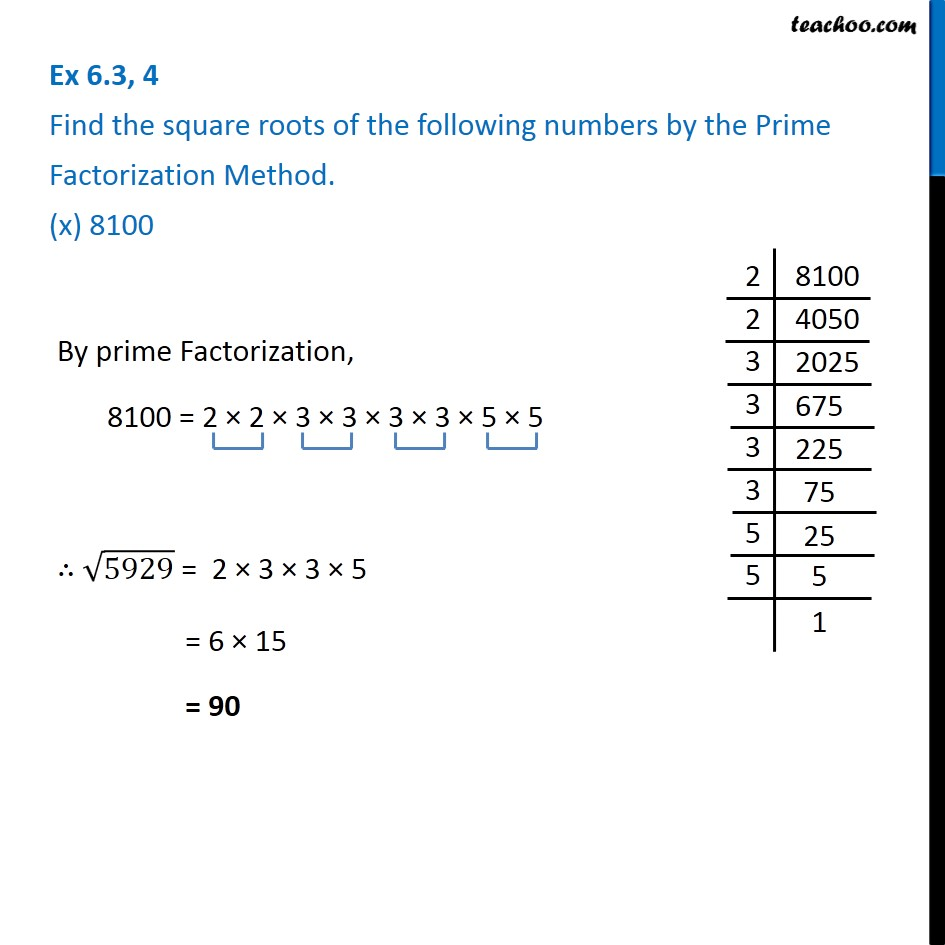Ex 6.3

Chapter 6 Class 8 Squares and Square Roots
Serial order wiseX`

Introducing your new favourite teacher - Teachoo Black, at only ₹83 per month

### Transcript

Ex 6.3, 4 Find the square roots of the following numbers by the Prime Factorization Method. (x) 8100 By prime Factorization, 8100 = 2 × 2 × 3 × 3 × 3 × 3 × 5 × 5 ∴ √5929 = 2 × 3 × 3 × 5 = 6 × 15 = 90